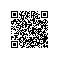# 如何在程序中产生概率性的结果

1，建立一个BitSet，size设为100。然后随机产生X个下标，然后将这些下标对应的位置设为true。

2，每次调用该方法时，再产生一个随机数作为下标，然后以此下标取得bitset中指定位置的值，返回。

1. private List<Integer> makeRndNumbers(int count, int boundry) {
2.     TreeSet<Integer> set = new TreeSet<Integer>();
3.     while(set.size() < count) {
5.     }
6.
7.     return new ArrayList<Integer>(set);

假设这个类命名为PercentChoice，其构造方法如下，这里使用了SecureRandom类，这是Java内置的一个安全的随机数发生器。

1. public PercentChoice(int percentage) {
2.     try {
3.         secRandom = SecureRandom.getInstance("SHA1PRNG");
4.         secRandom.setSeed(System.currentTimeMillis());
5.     } catch (NoSuchAlgorithmException e) {
6.         e.printStackTrace();
7.     }
8.
9.     for(Integer i : makeRndNumbers(percentage, 100)) {
10.         bitSet.set(i, true);
11.     }

1. public boolean getOneChoice() {
2.     return bitSet.get(Math.abs(secRandom.nextInt()) % 100);

1. public static void main(String[] args) throws InterruptedException {
2.     PercentChoice pc = new PercentChoice(20);
3.     int hits = 0;
4.     int times = 10000;
5.     for(int i = 0; i < times; i++) {
6.         if(pc.getOneChoice()) {
7.             hits++;
8.         }
9.     }
10.     System.out.println("Hits percentage " + (double)hits * 100 / (double)times + " %");